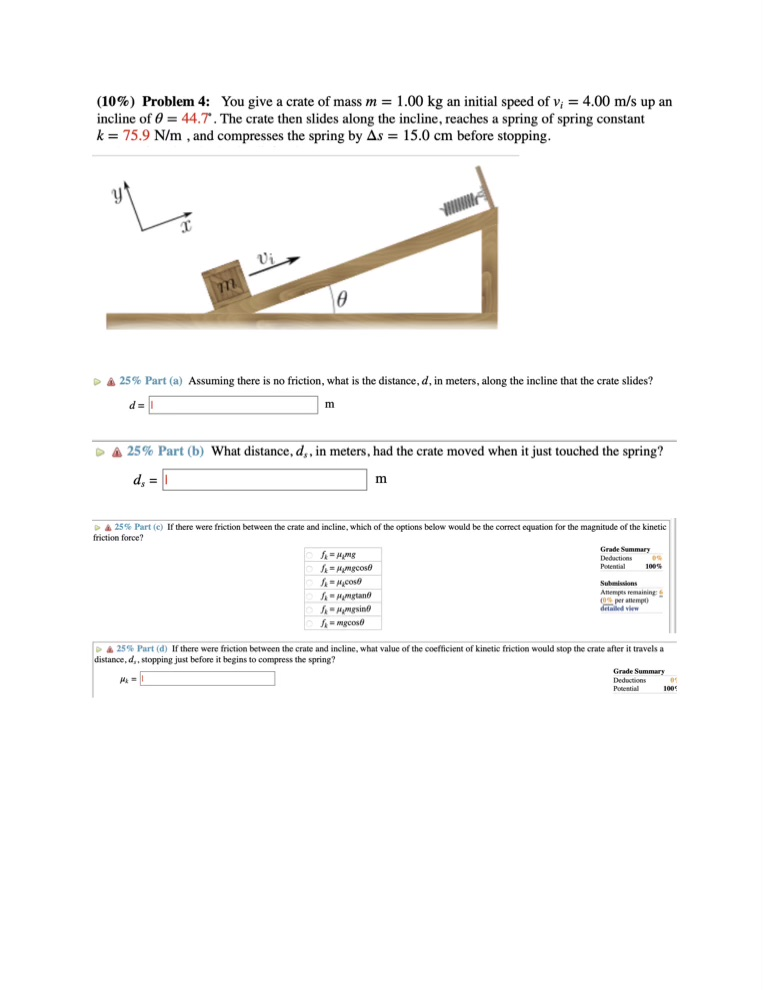# (Solved): Please help solve these physics problems with how-to steps to clarify and study (10\%) Problem 4: Yo ...(10\%) Problem 4: You give a crate of mass an initial speed of up an incline of . The crate then slides along the incline, reaches a spring of spring constant , and compresses the spring by before stopping. A Part (a) Assuming there is no friction, what is the distance, , in meters, along the incline that the crate slides? Part (b) What distance, , in meters, had the crate moved when it just touched the spring? A Part (c) If there were friction between the crate and incline, which of the options below woald be the correct equation for the magnitude of the kinetic friction force? i Part (d) If there were friction between the crate and incline, what value of the coefficient of kinetic friction would stop the crate after it travels a distance, , stopping just before it begins to compress the spring? Grade Summary Dedections Poeential 1005

We have an Answer from Expert